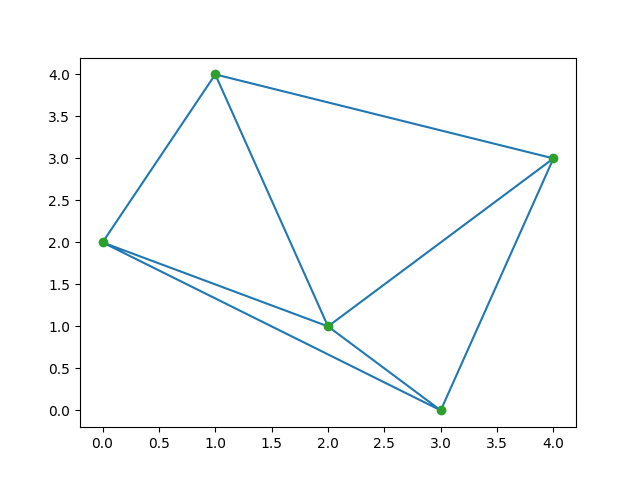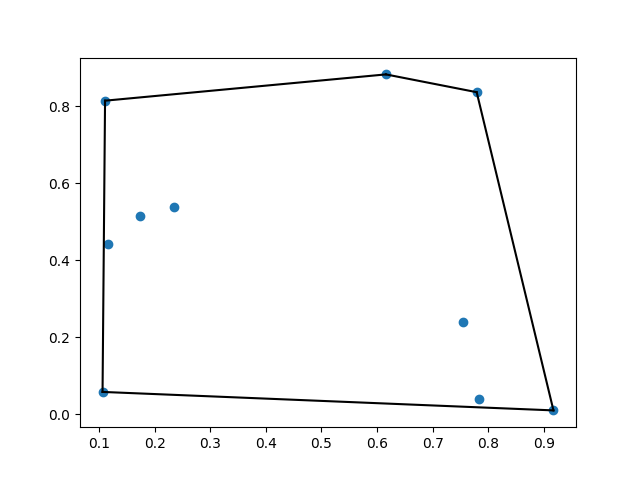GeeksforGeeks App
Open AppBrowser
Continue

# scipy.spatial – Spatial data structures and algorithms

In this article, we are going to see spatial data structure and algorithms, it is used to represent data in a geometric space.

## What is spatial data structure?

The spatial package computes the triangulations, Voronoi diagrams, and convex hulls of a set of points, by leveraging the Qhull library. Moreover, it contains KDTree implementations for nearest neighbor point queries and utilities for distance computations in various metrics.

### Example 1: Delaunay Triangulations

In mathematics and computational geometry, a Delaunay triangulation for a given set p of discrete points, in a plane is a triangulation DT(p) such that no point p is inside the circumcircle of any triangle in DT(p).

## Python

 `from` `scipy.spatial ``import` `Delaunay``import` `numpy as np``import` `matplotlib.pyplot as plt`` ` `points ``=` `np.array([[``1``, ``4``], [``2``, ``1``], [``3``, ``0``], ``                   ``[``0``, ``2``], [``4``, ``3``]])``tri ``=` `Delaunay(points)`` ` `plt.triplot(points[:, ``0``], points[:, ``1``], tri.simplices.copy())``plt.plot(points[:, ``0``], points[:, ``1``], ``'o'``)``plt.show()`

Output:### Example 2: Coplanar points

Coplanar points are three or more points that lie in the same plane. Recall that, a plane is a flat surface, which extends without end in all directions.

## Python

 `from` `scipy.spatial ``import` `Delaunay``import` `numpy as np`` ` `points ``=` `np.array([[``0``, ``0``], [``0``, ``1``], [``1``, ``0``], ``                   ``[``1``, ``1``], [``1``, ``1``]])``tri ``=` `Delaunay(points)``print``(tri.simplices)``print``(tri.coplanar)`

Output:

```[[3 1 0]
[2 3 0]]
[[4 0 3]]```

### Example 3: Convex Hulls

The convex hull or convex envelope of a set of points X in the euclidean space(or, more generally in affine space over the reals) is the smallest convex set that contains X.

## Python

 `from` `scipy.spatial ``import` `ConvexHull``import` `numpy as np``import` `matplotlib.pyplot as plt`` ` `points ``=` `np.random.rand(``10``, ``2``)``hull ``=` `ConvexHull(points)`` ` `plt.plot(points[:, ``0``], points[:, ``1``], ``'o'``)``for` `simplex ``in` `hull.simplices:``    ``plt.plot(points[simplex, ``0``], points[simplex, ``1``], ``'k-'``)`` ` `plt.show()`

Output:### Example 4: KPTrees

kd-tree is a quick nearest-neighbor lookup. And Kdtree() methods return the kd-tree object

## Python3

 `from` `scipy.spatial ``import` `KDTree`` ` `points ``=` `np.random.rand(``10``, ``2``)``kdtree ``=` `KDTree(points)``result ``=` `kdtree.query((``1``, ``1``))``print``(result)`

Output:

`(0.5144859720297681, 9)`

My Personal Notes arrow_drop_up